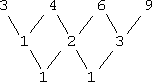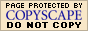Finding the Next Number in a Sequence:
General Examples
(page 4 of 7)

Sections: Common differences, Recursions, General examples, Non-math "sequences"What follows are just some additional examples, given so you can see the process at work.

• Find the missing number in the sequence:  3, 4, 6, 9, ___, 18.

First, I'll see if anything happens to pop out at me. To multiply from 3 to 4, I'd have to multiply by 4/3, but (4)(4/3) does not equal 6, so that must not be the rule. To add from 3 to 4, I'd have to add 1, but 4 + 1 is not 6; 4 + 2 = 6. Wait....

3 + 1 = 4
4 + 2 = 6

6 + 3 = 9

Hmm... What if the rule is "add the next bigger number to the last term"? Then I'd have:

9 + 4 = 13

Does this fit? Do I get "18" for the next value?

13 + 5 = 18

Yup; it worked! So it would appear that the rule is "add the next bigger number to the previous term", and:   Copyright © Elizabeth Stapel 2002-2011 All Rights Reserved

The missing number is 13.

Note that I could have gone straight to the differences:Since the second differences are the same, then the formula is a quadratic. Plugging in the first three data points, I get:

a + b + c = 3
4a + 2b + c = 4
9a + 3b + c = 6

Solving this system of equations, I get:

an = 0.5n2 − 0.5n + 3

Plugging in n = 5 for the missing fifth term, I get:

a5 = 0.5(25) − 0.5(5) + 3 = 12.5 − 2.5 + 3 = 10 + 3 = 13

So my previous answer was right, or has at least been confirmed as logical.

• Find the formula for the n-th term in the sequence:  4, 12, 20, 28, 36,...

To add from 4 to 12, I'd have to use 8. To add from 12 to 20, I'd also have to use 8. Let's check to see if "add 8" is the rule:

4 + 8 = 12
12 + 8 = 20

20 + 8 = 28

28 + 8 = 36

It appears that the rule is "add 8". So what is the rule for the n-th term? Let's look at the terms:

n = 1:  4
n = 2:  4 + 8

n = 3:  4 + 8 + 8 = 4 + 2×8

n = 4:  4 + 8 + 8 + 8 = 4 + 3×8

n = 5:  4 + 8 + 8 + 8 + 8 = 4 + 4×8

Following this pattern, the rule for the n-th term will be:

an = 4 + (n − 1)8

<< Previous  Top  |  1 | 2 | 3 | 4 | 5 | 6 | 7  |  Return to Index  Next >>

 Cite this article as: Stapel, Elizabeth. "Sequences: General Examples." Purplemath. Available from     https://www.purplemath.com/modules/nextnumb4.htm. Accessed [Date] [Month] 2016

MathHelp.com Courses
This lesson may be printed out for your personal use.